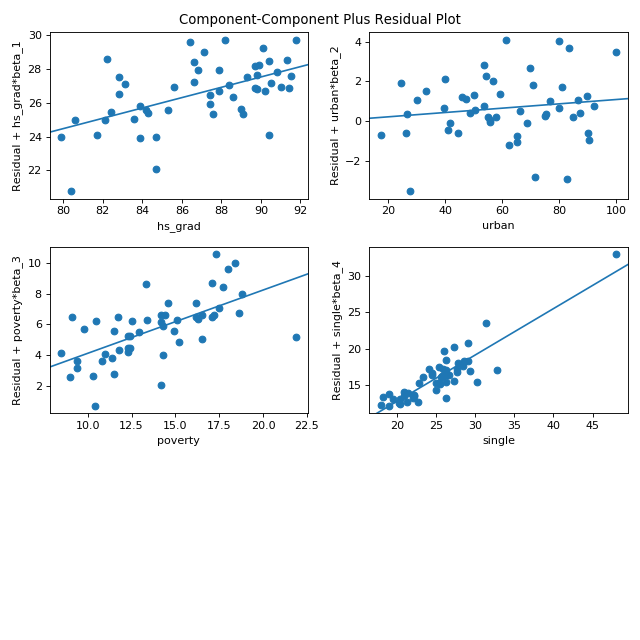statsmodels.graphics.regressionplots.plot_ccpr_grid¶

statsmodels.graphics.regressionplots.plot_ccpr_grid(results, exog_idx=None, grid=None, fig=None)[source]

Generate CCPR plots against a set of regressors, plot in a grid.

Generates a grid of CCPR (component and component-plus-residual) plots.

Parameters
resultsresult instance

uses exog and params of the result instance

exog_idxNone or list of int

(column) indices of the exog used in the plot

gridNone or tuple of int (nrows, ncols)

If grid is given, then it is used for the arrangement of the subplots. If grid is None, then ncol is one, if there are only 2 subplots, and the number of columns is two otherwise.

figMatplotlib figure instance, optional

If given, this figure is simply returned. Otherwise a new figure is created.

Returns
figMatplotlib figure instance

If ax is None, the created figure. Otherwise the figure to which ax is connected.

plot_ccpr

Creates CCPR plot for a single regressor.

Notes

Partial residual plots are formed as:

Res + Betahat(i)*Xi versus Xi

Betahat(i)*Xi versus Xi

References

Examples

Using the state crime dataset seperately plot the effect of the each variable on the on the outcome, murder rate while accounting for the effect of all other variables in the model.

>>> import statsmodels.api as sm
>>> import matplotlib.pyplot as plt
>>> import statsmodels.formula.api as smf
>>> fig = plt.figure(figsize=(8, 8))